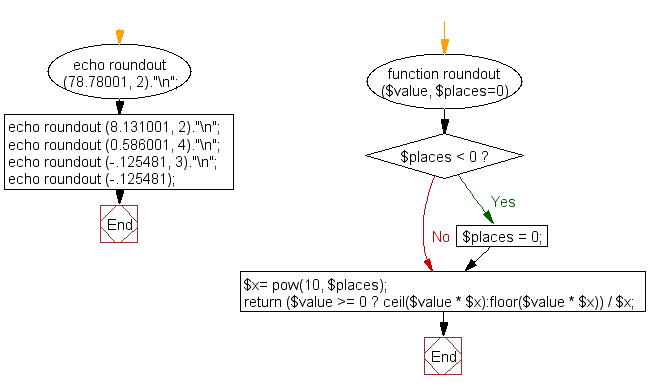﻿ PHP Math Exercise: Round a float away from zero to a specified number of decimal places - w3resource# PHP Math Exercises: Round a float away from zero to a specified number of decimal places

## PHP math: Exercise-8 with Solution

Write a PHP function to round a float away from zero to a specified number of decimal places.

Sample Data :
(78.78001, 2)
(8.131001, 2)
(0.586001, 4)
(-.125481, 3)
-.125481

Sample Solution:

PHP Code:

``````<?php
function roundout (\$value, \$places=0) {
if (\$places < 0) { \$places = 0; }
\$x= pow(10, \$places);
return (\$value >= 0 ? ceil(\$value * \$x):floor(\$value * \$x)) / \$x;
}
echo roundout (78.78001, 2)."\n";
echo roundout (8.131001, 2)."\n";
echo roundout (0.586001, 4)."\n";
echo roundout (-.125481, 3)."\n";
echo roundout (-.125481);
?>
```
```

Sample Output:

```78.79
8.14
0.5861
-0.126
-1
```

Flowchart :PHP Code Editor:

Have another way to solve this solution? Contribute your code (and comments) through Disqus.

What is the difficulty level of this exercise?

﻿

## PHP: Tips of the Day

PHP represents "no value" with the null keyword. It's somewhat similar to the null pointer in C-language and to the NULL value in SQL.

Setting the variable to null:

Example:

```\$nullvar = null; // directly
function doSomething() {} // this function does not return anything
\$nullvar = doSomething(); // so the null is assigned to \$nullvar
```

Checking if the variable was set to null:

```if (is_null(\$nullvar)) { /* variable is null */ }
if (\$nullvar === null) { /* variable is null */ }
```

Null vs undefined variable

If the variable was not defined or was unset then any tests against the null will be successful but they will also generate a Notice: Undefined variable: nullvar:

```\$nullvar = null;
unset(\$nullvar);
if (\$nullvar === null) { /* true but also a Notice is printed */ }
if (is_null(\$nullvar)) { /* true but also a Notice is printed */ }
```

Therefore undefined values must be checked with isset:

```if (!isset(\$nullvar)) { /* variable is null or is not even defined */ }
```